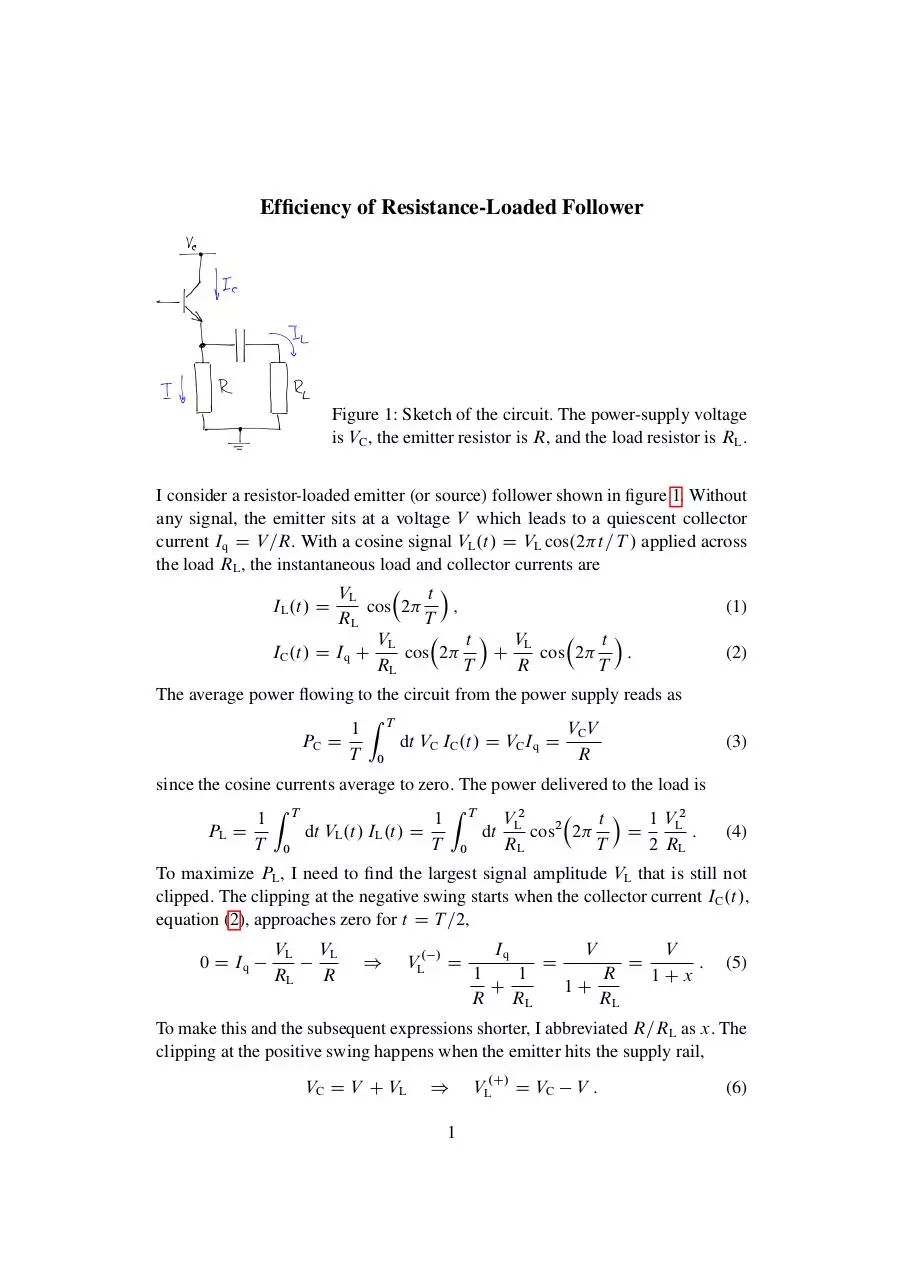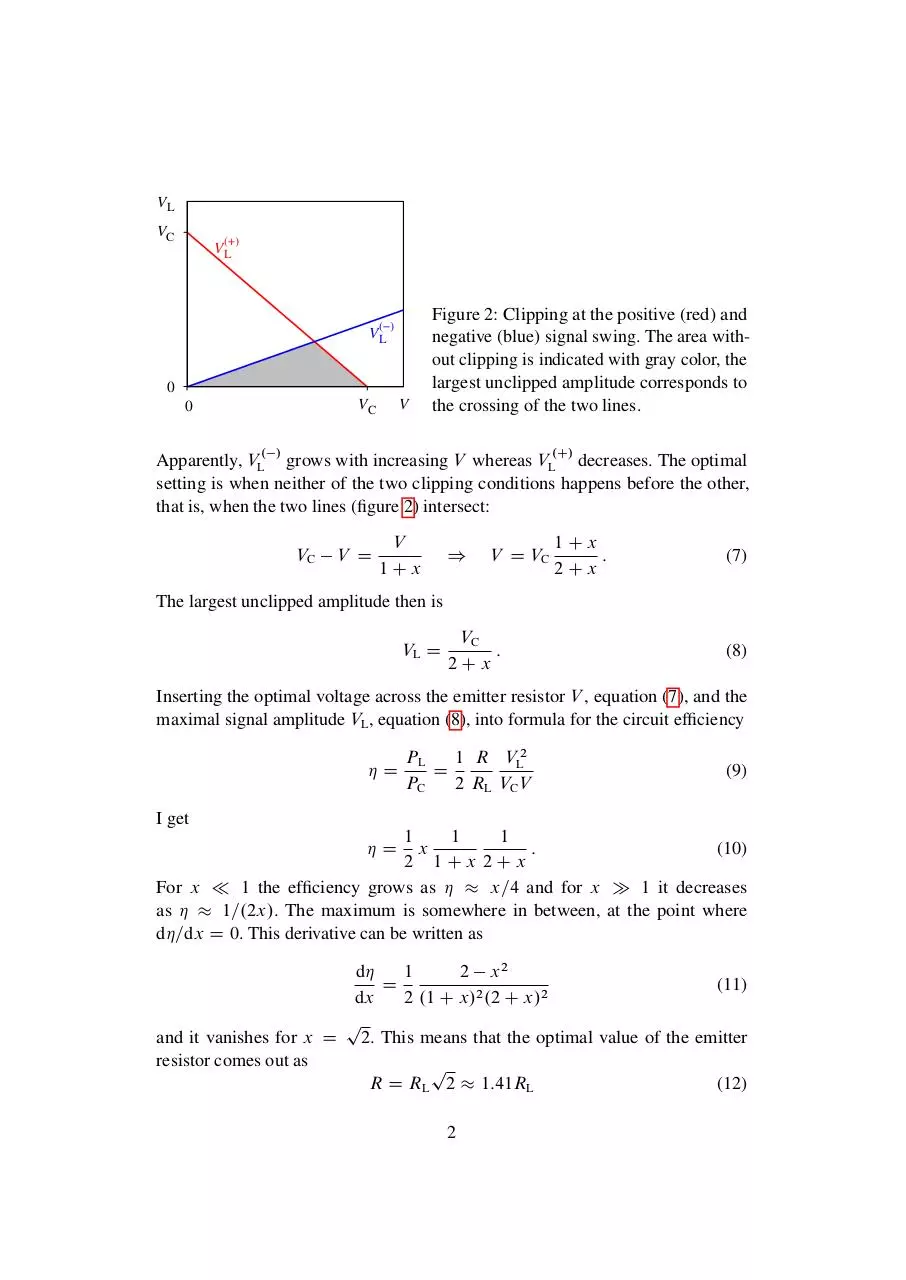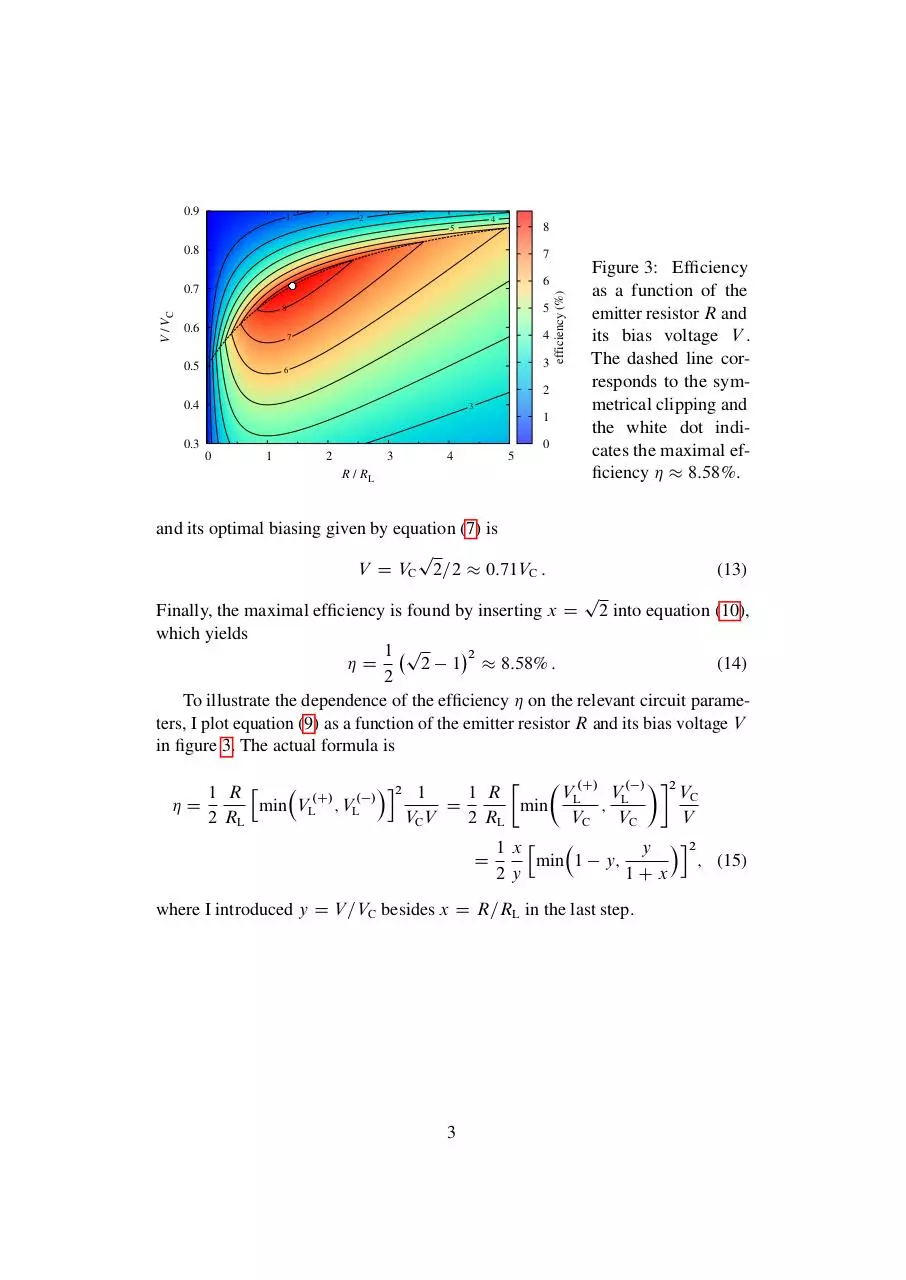### File information

Author: Jindrich Kolorenc, http://orcid.org/0000-0003-2627-8302

This PDF 1.5 document has been generated by LaTeX with hyperref package / pdfTeX-1.40.17, and has been sent on pdf-archive.com on 11/05/2017 at 16:54, from IP address 147.231.x.x. The current document download page has been viewed 395 times.
File size: 208.11 KB (3 pages).
Privacy: public file

### Document preview

Figure 1: Sketch of the circuit. The power-supply voltage
is VC , the emitter resistor is R, and the load resistor is RL .
I consider a resistor-loaded emitter (or source) follower shown in figure 1. Without
any signal, the emitter sits at a voltage V which leads to a quiescent collector
current Iq D V =R. With a cosine signal VL .t/ D VL cos.2 t=T / applied across
 t
VL
IL .t/ D
cos 2
;
(1)
RL
T
 t V
 t
VL
L
IC .t/ D Iq C
cos 2
C
cos 2
:
(2)
RL
T
R
T
The average power flowing to the circuit from the power supply reads as
Z
1 T
VC V
PC D
dt VC IC .t/ D VC Iq D
T 0
R
since the cosine currents average to zero. The power delivered to the load is
Z
Z
 t  1 V2
1 T VL2
1 T
L
PL D
dt VL .t/ IL .t/ D
dt
cos2 2
D
:
T 0
T 0
RL
T
2 RL

(3)

(4)

To maximize PL , I need to find the largest signal amplitude VL that is still not
clipped. The clipping at the negative swing starts when the collector current IC .t/,
equation (2), approaches zero for t D T =2,
0 D Iq

VL
RL

VL
R

)

VL.

/

D

Iq
V
V
D
D
:
1
1
R
1Cx
C
1C
R
RL
RL

(5)

To make this and the subsequent expressions shorter, I abbreviated R=RL as x. The
clipping at the positive swing happens when the emitter hits the supply rail,
VC D V C VL

)
1

VL.C/ D VC

V:

(6)

VL
VC

V(+)
L

V(−)
L
0
V

VC

0

Figure 2: Clipping at the positive (red) and
negative (blue) signal swing. The area without clipping is indicated with gray color, the
largest unclipped amplitude corresponds to
the crossing of the two lines.

Apparently, VL. / grows with increasing V whereas VL.C/ decreases. The optimal
setting is when neither of the two clipping conditions happens before the other,
that is, when the two lines (figure 2) intersect:
VC

V D

V
1Cx

)

V D VC

1Cx
:
2Cx

(7)

The largest unclipped amplitude then is
VL D

VC
:
2Cx

(8)

Inserting the optimal voltage across the emitter resistor V , equation (7), and the
maximal signal amplitude VL , equation (8), into formula for the circuit efficiency
D

PL
1 R VL2
D
PC
2 RL VC V

(9)

I get
1
1
1
x
:
(10)
2 1Cx 2Cx
For x  1 the efficiency grows as   x=4 and for x  1 it decreases
as   1=.2x/. The maximum is somewhere in between, at the point where
d=dx D 0. This derivative can be written as
D

d
1
2 x2
D
(11)
dx
2 .1 C x/2 .2 C x/2
p
and it vanishes for x D 2. This means that the optimal value of the emitter
resistor comes out as
p
R D RL 2  1:41RL
(12)
2

0.9

1

2

4

8

5

0.8

7

V / VC

0.7

5

8

0.6

4

7

0.5

3

6

2
0.4

3

1
0.3

0
0

1

2

3

4

5

R / RL

efficiency (%)

6

Figure 3: Efficiency
as a function of the
emitter resistor R and
its bias voltage V .
The dashed line corresponds to the symmetrical clipping and
the white dot indicates the maximal efficiency   8:58%.

and its optimal biasing given by equation (7) is
p
V D VC 2=2  0:71VC :

(13)

p
Finally, the maximal efficiency is found by inserting x D 2 into equation (10),
which yields
2
1 p
D
2 1  8:58% :
(14)
2
To illustrate the dependence of the efficiency  on the relevant circuit parameters, I plot equation (9) as a function of the emitter resistor R and its bias voltage V
in figure 3. The actual formula is

 .C/ . / 2
i2 1

1 R h
1 R
V
V
VC
. /
.C/
D
min VL ; VL
D
min L ; L
2 RL
VC V
2 RL
VC
VC
V

1xh
y i2
D
min 1 y;
; (15)
2y
1Cx
where I introduced y D V =VC besides x D R=RL in the last step.

3### Share on social networks

#### HTML Code

Copy the following HTML code to share your document on a Website or Blog